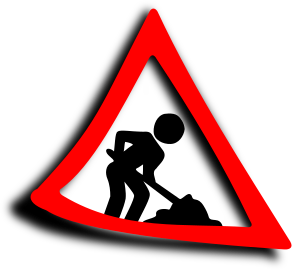Stepping Stones...

How ? Just how does the Megaprocessor work ?

I've built the Megaprocessor so that we can see how a microprocessor works. We can see all the components and how they're arranged and we can see the electrical/logic signals as they change. But what is happening ? If you've never encountered a transistor before or have only heard of logic from Mr Spock then it may require quite a big leap of intuition to understand what you're looking at. On this page is a series of short videos that will break down that big leap into a series of smaller more manageable steps.UNDER CONSTRUCTION : The videos are first attempts and may be a bit rough.

These can be watched here or through this YouTube playlist.

• Step 1 : Volts and Amps - <<TODO>>

• Step 2 : The Transistor - The transistor is the key component of the Megaprocessor and indeed of all modern processors. The fundamental operation of a transistor is to amplify current. Here we see one doing that. We then see how it can act as a switch and how that can then lead on to carrying out the operations required for a computer.

• Step 3 : Two Transistors, Part 1 - There are two ways that two transistors can be connected together. Here we look at one of those ways (in series) and see what the circuit can do.

• Step 4 : Logic - This is where we start to look at logic and the different functions that are available. We see how we should interpret the two transistor circuit we saw previously.

• Step 5 : Two Transistors, Part 2 - And here we look at the second way that two transistors can be connected (in parallel) and see what the circuit can do.

• Step 6 : Many Transistors - Here we look at how we can combine the simple circuits we've seen so far to create a new function using 6 transistors. This will be the Exclusive Or (XOR) function which is useful for arithmetic, which we will be looking at next. After this we will only be dealing with logic and will no longer consider the electronics that implements it.

• Step 7 : Numbers & Arithmetic - Here we look at how computers represent numbers, i.e. the binary system. We then show how the simple logic functions we have so far can be used to add numbers.

• Step 8 : Time & Memory - So far the logic we've looked at allows us to combine some input signals to generate an output. But processors carry out a sequence of operations so we must add the concepts of time and memory.
<<TODO>>
• Step 9 : The State Machine - The operation of a processor is regulated by "state machines". We now have enough to create our first and we shall use it to control a pedestrian crossing.
<<TODO>>
• Step 10 ++ : << TODO >> there will be maybe another 6-10 steps to get to a processor